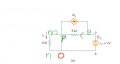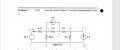# Are there any simple and standralized ways to count the number of meshes and loops in a circuit?

#### Timey

Joined Dec 6, 2020
4
In this example,mn is short circuit,and we have a simple circuit,where I can easily count the numbe of mesh (or loop?) by randoml way and its 2 in this pariticular case, p----g、a-g-p-o-b.What if I encounter a more complicated one such as the one shown below,how should I count it ,are there and standralized procedures for doing it ???#### WBahn

Joined Mar 31, 2012
29,184
You talk like you are showing two circuits but your post only has one attached.

What is mesh p----g mean?

Are you sure that m and n are shorted? Usually there is a connection dot on nodes that cross if they are connected. But, this isn't always the case. How does your text represent wires that cross but don't connect? Does one appear to jump over the other?

If the circuit is planar (i.e., can be drawn on a sheet of paper without any branches crossing) then counting meshes is a simple matter of counting the number of "panes" shown (think of a window that is separated into multiple panes). In the circuit you have shown, there are three meshes. You can simplify the circuit to analyze it since the 4 Ω resistor is shorted. But if you do this, then you can remove the dependent current source as well, since Ix is identically zero. Hence it can be reduced to a single loop having two components, a 1 V source and a 5 Ω resistor. But the circuit given still has three meshes.

#### Timey

Joined Dec 6, 2020
4
You talk like you are showing two circuits but your post only has one attached.

What is mesh p----g mean?

Are you sure that m and n are shorted? Usually there is a connection dot on nodes that cross if they are connected. But, this isn't always the case. How does your text represent wires that cross but don't connect? Does one appear to jump over the other?

If the circuit is planar (i.e., can be drawn on a sheet of paper without any branches crossing) then counting meshes is a simple matter of counting the number of "panes" shown (think of a window that is separated into multiple panes). In the circuit you have shown, there are three meshes. You can simplify the circuit to analyze it since the 4 Ω resistor is shorted. But if you do this, then you can remove the dependent current source as well, since Ix is identically zero. Hence it can be reduced to a single loop having two components, a 1 V source and a 5 Ω resistor. But the circuit given still has three meshes.
sorry,i missed some photo,I mean mesh p----g---p..Another image of more complicated circuit is shown below#### WBahn

Joined Mar 31, 2012
29,184
You've labeled every corner or intersection in the diagram, but not all of them. For instance, if the corners above and below the 12 V source and 2 A source deserve labels, why don't the corners to the left and right of the 4 A source deserve them?

Much more valuable than labeling every corner or intersection is to label every node. Labels 'f', a', 'c', and 'm' are all on the same node, so just assign a single node label. The same for labels 'd' and 'h'.

Even more valuable is to identify the "essential nodes", which are the nodes connected to more than two components (and, consequently, the nodes that don't have trivial solutions to Kirchhoff's Current Law).

•Timey

#### Timey

Joined Dec 6, 2020
4
right,thanks. plus,I still wanna know how to count the number of meshes ," counting meshes is a simple matter of counting the number of "panes" shown (think of a window that is separated into multiple panes" what does it mean?? how do I count the number of panes in the second image??

#### ericgibbs

Joined Jan 29, 2010
18,072
hi T,
Does this help.?
E